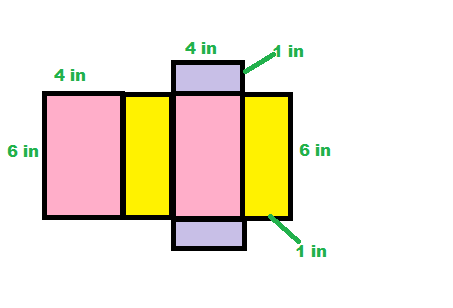# Surface Area Lesson for Kids

Instructions:

question 1 of 3

### Lucas is going to find the surface area of a board game box. He needs to find the area for how many sides before calculating surface area?

Create Your Account To Take This Quiz

As a member, you'll also get unlimited access to over 79,000 lessons in math, English, science, history, and more. Plus, get practice tests, quizzes, and personalized coaching to help you succeed.

Try it risk-free for 30 days. Cancel anytime

### 1. Find the surface area of this box.### 2. What is surface area?

Create your account to access this entire worksheet
Quizzes, practice exams & worksheets
Certificate of Completion
Create an account to get started

Finding the surface area will be the subject of this quiz and worksheet. You will need to figure out how to determine the surface area of different objects, and also give a definition of surface area.

## Quiz & Worksheet Goals

For this quiz, you should know:

• The meaning of surface area
• How to find the surface area of rectangular objects
• The surface area of a cube
• The number of sides found on a board game box

## Skills Practiced

By using this quiz and worksheet, you can practice these skills:

• Reading comprehension - make sure you understand the most important information from the lesson on surface area
• Defining key concepts - give a definition for surface area
• Problem solving - use what you know to solve surface area math problems

Check out the lesson called Surface Area Lesson for Kids if you want to find out more. After studying this lesson, you should have no trouble performing the following:

• Examining all the sides of a rectangular shape
• Figuring out the surface area of shapes
• Knowing the difference between the narrow, top and bottom parts of a box
Final ExamMath for Kids
Status: Not Started
Chapter ExamGeometry for Elementary School
Status: Not Started

Support